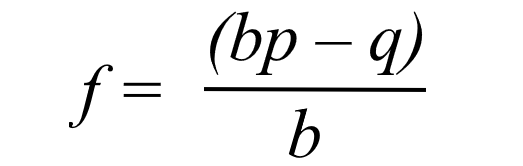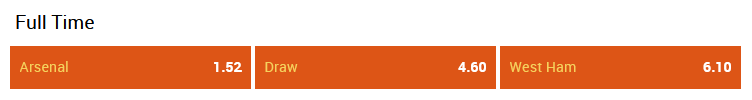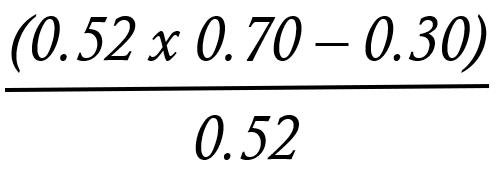# Kelly Bet – Explained with Examples

To help improve your betting bankroll management, you want to use a proven strategy – the Kelly Criterion, AKA the Kelly Bet, is just that. This page will explain all you need to know about the Kelly Criterion, so you can put it to work for you. Once you understand the formula, placing your Kelly Bets will only take a few seconds.

## What is the Kelly Criterion?

In a nutshell, the Kelly Criterion is nothing more than a mathematical formula that calculates what percentage of your funds should be allocated to a particular bet (or investment). It uses the perceived win/loss probabilities combined with the price of the bet to determine value in the market.

This is the formula for the Kelly Bet.When looking at the Kelly Bet for the first time, it does appear a little complicated but it’s actually super-simple. You only need three values and a calculator.

• f = this is the result of the equation. It will tell us how much of our bankroll to bet (if any at all).
• b = the decimal odds -1.
• p = the probability of your bet selection winning (being correct).
• q = the probability of your bet selection losing (being incorrect).

The value of b is out of the punter’s control as the bookmaker sets the odds. However, for the Kelly Bet to be effective over the course of a season, you must accurately predict the probability of p. If you are wide of the with what you see as the perceived probability, you will not get accurate results from the Kelly Bet formula.

## Kelly Bet Explained

The Kelly Bet is merely a staking system; therefore, the fundamentals of your bet selection will remain the same. You will only bet when there is value in the market. For those who are new to betting online, we recommend taking a look at our EPL predictions page for some solid betting tips.

Let’s look at some examples to help explain the Kelly Criterion.

Football: English Premier League (19-09-2020)This is the second game of the Premier League season. Arsenal won their opener, 0-3, away at Fulham, while West Ham lost 2-0 at home to Newcastle. Based on the betting odds – taken from 888sport – the match result probabilities are:

• Arsenal win: 65.7%
• Draw: 21.7%
• West Ham 16.4%
• These percentages include the bookmaker’s margin, which is why the total is higher than 100%.

After doing our research, we feel that Arsenal have a 70% chance of winning (that is our perceived probability), so let’s put the odds and our probability into the Kelly Bet formula.

• b = the decimal odds: 1.52 -1 = 0.52
• p = the probability of your bet selection winning (being correct). 70% of 1 = 0.70
• q = the probability of your bet selection losing (being incorrect). 30% of 1 = 0.30After doing the equation, we know that f = 0.12307692307. Multiply this by 100 = 12.3%. That is the recommended amount of your bankroll to stake according to the Kelly Bet formula. If f equals a negative number, then you should not bet, as there is no value.

For instance, let’s assume that Arsenal have a 50% probability of winning.

### (0.52 (b) x 0.50 (p) – 0.50 (q)) ÷ 0.50 (b)

The result now is, f = -0.461538. No bet because of the negative value. The odds are not high enough for perceived risk.

### Summary

Warren Buffet, one of the world’s richest men, is known to use the Kelly Criterion for investing. So, if it’s good enough for him, it’s good enough for us.

As pointed out, the most important part is the accuracy of your win probability. You can simply use the bookmaker’s percentages if you choose, but ideally, you want to find extra value in the market. Secondly, this method also highlights the importance of finding the best odds. This also effects the stake, and the payout when you win.